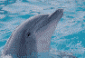# summary(mxRun(myMxModel)) gives strange results

1 post / 0 newOffline
Joined: 10/19/2009 - 15:38
summary(mxRun(myMxModel)) gives strange results
AttachmentSizecorrectLikelihood.R6.16 KB

(1) run the attached. (NOTE: data sets at the beginning should be into R matrix objects ObsCovMZ and ObsCovDZ). then examine the result of "summary(test2)." i get (after the parameters):

Observed statistics: 0
Estimated parameters: 45
Degrees of freedom: -45
-2 log likelihood: 29434.03
Saturated -2 log likelihood:
Chi-Square:
p:
AIC (Mx):
BIC (Mx):
adjusted BIC:
RMSEA:

(2) suggest placing the chi square and contribution to chi square for each group in the MxModel object, so users can access these quantities directly.

(3) would it be useful for OpenMx to note intraclass relationships and calculate the correct df for the chi square?